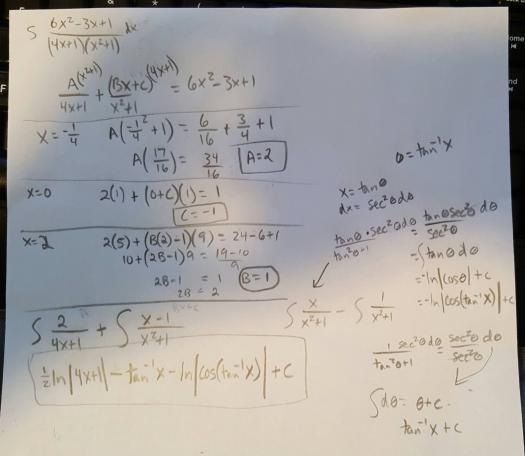# check my solution: $\displaystyle \int \dfrac{(6x^2 - 3x + 1) dx}{(4x + 1)(x^2 + 1)}$

2 posts / 0 new
steph00
check my solution: $\displaystyle \int \dfrac{(6x^2 - 3x + 1) dx}{(4x + 1)(x^2 + 1)}$

hey...can anyone here check my solution to the problem??not sure whether I got it correctly and do not want to pay https://www.chegg.com/ or http://yourhomeworkhelp.org for the thing I practically did myselfthanks

Jhun Vert$\displaystyle \int \dfrac{6x^2 - 3x + 1}{(4x + 1)(x^2 + 1)} dx$

$\dfrac{6x^2 - 3x + 1}{(4x + 1)(x^2 + 1)} = \dfrac{A}{4x + 1} + \dfrac{Bx + C}{x^2 + 1}$

$6x^2 - 3x + 1 = A(x^2 + 1) + (Bx + C)(4x + 1)$

$6x^2 - 3x + 1 = A(x^2 + 1) + B(4x^2 + x) + C(4x + 1)$

Set x = 0
$A + C = 1$   ←   Eq. (1)

Equate coefficients of x
$B + 4C = -3$   ←   Eq. (2)

Equate coefficients of x2
$A + 4B = 6$   ←   Eq. (3)

From Eq. (1), Eq. (2), and Eq. (3)
$A = 2$
$B = 1$
$C = -1$

Therefore,
$\displaystyle \int \dfrac{6x^2 - 3x + 1}{(4x + 1)(x^2 + 1)} dx = \int \left( \dfrac{2}{4x + 1} + \dfrac{x - 1}{x^2 + 1} \right) \, dx$

$\displaystyle = 2\int \dfrac{dx}{4x + 1} + \int \dfrac{x \, dx}{x^2 + 1} - \int \dfrac{dx}{x^2 + 1}$

$\displaystyle = \dfrac{2}{4} \int \dfrac{4 \, dx}{4x + 1} + \dfrac{1}{2} \int \dfrac{2x \, dx}{x^2 + 1} - \int \dfrac{dx}{x^2 + 1}$

$= \frac{1}{2} \ln (4x + 1) + \frac{1}{2} \ln (x^2 + 1) - \arctan x + C$       answer

• Mathematics inside the configured delimiters is rendered by MathJax. The default math delimiters are $$...$$ and $...$ for displayed mathematics, and $...$ and $...$ for in-line mathematics.